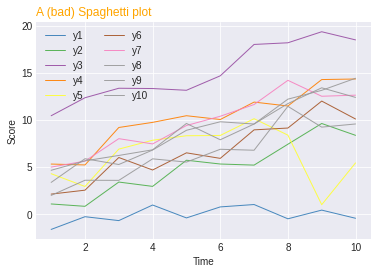# Spaghetti Plot

A spaghetti plot is a line plot with many lines displayed together. The problem of a spaghetti plot is that it is really hard to read, and thus provides few insights about the data. You can find a good documentation here. This post explains how to realise it with python and, more importantly, provide a few propositions to make it better.

## Spaghetti plot code

``````# libraries
import matplotlib.pyplot as plt
import numpy as np
import pandas as pd

# Make a data frame
df=pd.DataFrame({'x': range(1,11), 'y1': np.random.randn(10), 'y2': np.random.randn(10)+range(1,11), 'y3': np.random.randn(10)+range(11,21), 'y4': np.random.randn(10)+range(6,16), 'y5': np.random.randn(10)+range(4,14)+(0,0,0,0,0,0,0,-3,-8,-6), 'y6': np.random.randn(10)+range(2,12), 'y7': np.random.randn(10)+range(5,15), 'y8': np.random.randn(10)+range(4,14), 'y9': np.random.randn(10)+range(4,14), 'y10': np.random.randn(10)+range(2,12) })

# Change the style of plot
plt.style.use('seaborn-darkgrid')

# Create a color palette
palette = plt.get_cmap('Set1')

# Plot multiple lines
num=0
for column in df.drop('x', axis=1):
num+=1
plt.plot(df['x'], df[column], marker='', color=palette(num), linewidth=1, alpha=0.9, label=column)

plt.legend(loc=2, ncol=2)

plt.title("A (bad) Spaghetti plot", loc='left', fontsize=12, fontweight=0, color='orange')
plt.xlabel("Time")
plt.ylabel("Score")

# Show the graph
plt.show()``````## Highlight a group

Let’s say you plot many groups, but the actual reason for that is to explain the feature of one particular group compared to the others.

Then a good practice is to highlight this group: make it appear different, and give it a proper annotation. Here, the behaviour of the orange line is obvious.

See the code here.

## Use small multiples

If all groups interest you, a good solution would be to split them in separate subplots. As you can see here, the behaviour of each group is much more readable than a spaghetti plot.

See the code of this version here.

## Small multiples (variant)

Another option is to do the same but display all the groups on each subplot discretely. It’s up to you to choose the version you prefer. Here is the code.

## Area chart

If you decide to use small multiples, I have a personal preference for using area charts instead of line plots. I find easier to see the trends in an area chart, but it is my personal opinion.

In any case, here is the code of this chart.

## Contact & Edit

👋 This document is a work by Yan Holtz. Any feedback is highly encouraged. You can fill an issue on Github, drop me a message onTwitter, or send an email pasting `yan.holtz.data` with `gmail.com`.